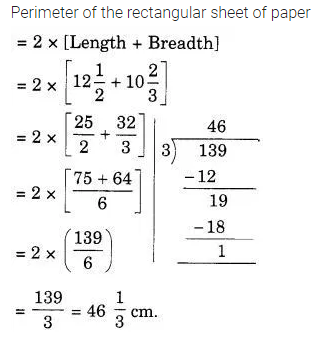# A rectangular sheet of paper is 12 1/2 cm long 10 2/3 cm wide. find its perimeter

A rectangular sheet of paper is 12$\frac { 1 }{ 2 }$ cm long 10$\frac { 2 }{ 3 }$ cm wide. find its perimeter